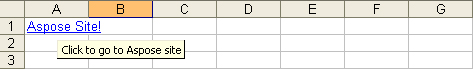Contents
[ ]

1. Set up the worksheet:
1. Instantiate an Application object. (VSTO only.)
3. Get the first sheet.
3. Save the document.

These steps are shown in the code examples below. The first examples shows how to use VSTO with either C# or Visual Basic to add a hyperlink to a cell. The examples that follow show how to do the same thing using Aspose.Cells for .NET, again using C# or Visual Basic.

The code samples generate an Excel file that has a hyperlink in cell A1 on the first worksheet.C#

`````` .......

using Microsoft.VisualStudio.Tools.Applications.Runtime;

using Excel = Microsoft.Office.Interop.Excel;

using Office = Microsoft.Office.Core;

using System.Reflection;

.......

//Instantiate the Application object.

Excel.ApplicationClass ExcelApp = new Excel.ApplicationClass();

//Get the First sheet.

Excel.Worksheet objSheet = (Excel.Worksheet)objBook.Sheets["Sheet1"];

//Define a range object(A1).

Excel.Range _range;

_range = objSheet.get_Range("A1", "A1");

//Save the excel file.

//Quit the Application.

ExcelApp.Quit();
``````

C#

`````` .......

using Aspose.Cells;

.......

//Instantiate a new Workbook object.

Workbook workbook = new Workbook();

//Get the First sheet.

Worksheet worksheet = workbook.Worksheets;

//Define A1 Cell.

Aspose.Cells.Cell cell = worksheet.Cells["A1"];